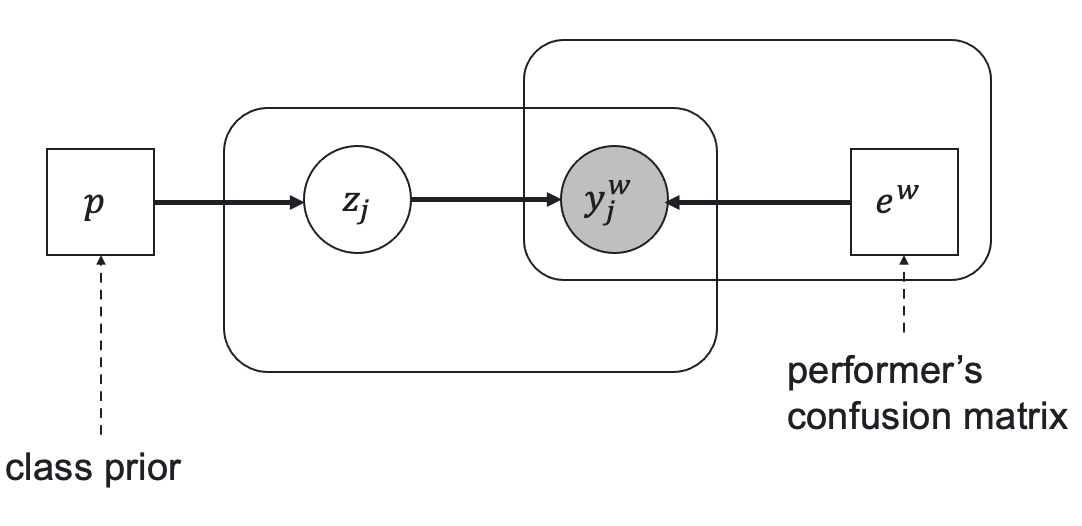• Overview
• Aggregations
• Aggregation
• Classification
• Embeddings
• Image segmentation
• SegmentationEM
• SegmentationMajorityVote
• SegmentationRASA
• Pairwise
• Texts
• ROVER
• TextHRRASA
• TextRASA
• TextSummarization
• utils
• Datasets
• Metrics
• Postprocessing

# DawidSkene

crowdkit.aggregation.classification.dawid_skene.DawidSkene | Source code

DawidSkene(
self,
n_iter: int = 100,
tol: float = 1e-05
)


Dawid-Skene aggregation model.

Probabilistic model that parametrizes workers' level of expertise through confusion matrices.

Let $e^w$ be a worker's confusion (error) matrix of size $K \times K$ in case of $K$ class classification, $p$ be a vector of prior classes probabilities, $z_j$ be a true task's label, and $y^w_j$ be a worker's answer for the task $j$. The relationships between these parameters are represented by the following latent label model.Here the prior true label probability is

$\operatorname{Pr}(z_j = c) = p[c],$

and the distribution on the worker's responses given the true label $c$ is represented by the corresponding column of the error matrix:

$\operatorname{Pr}(y_j^w = k | z_j = c) = e^w[k, c].$

Parameters $p$ and $e^w$ and latent variables $z$ are optimized through the Expectation-Maximization algorithm.

A. Philip Dawid and Allan M. Skene. Maximum Likelihood Estimation of Observer Error-Rates Using the EM Algorithm. Journal of the Royal Statistical Society. Series C (Applied Statistics), Vol. 28, 1 (1979), 20–28.

https://doi.org/10.2307/2346806

## Parameters Description

Parameters Type Description
n_iter int

The number of EM iterations.

labels_ Optional[Series]

Tasks' labels. A pandas.Series indexed by task such that labels.loc[task] is the tasks's most likely true label.

probas_ Optional[DataFrame]

Tasks' label probability distributions. A pandas.DataFrame indexed by task such that result.loc[task, label] is the probability of task's true label to be equal to label. Each probability is between 0 and 1, all task's probabilities should sum up to 1

priors_ Optional[Series]

A prior label distribution. A pandas.Series indexed by labels and holding corresponding label's probability of occurrence. Each probability is between 0 and 1, all probabilities should sum up to 1

errors_ Optional[DataFrame]

Workers' error matrices. A pandas.DataFrame indexed by worker and label with a column for every label_id found in data such that result.loc[worker, observed_label, true_label] is the probability of worker producing an observed_label given that a task's true label is true_label

Examples:

from crowdkit.aggregation import DawidSkene